CLAT  >  Test: Data Interpretation- 3

# Test: Data Interpretation- 3 - CLAT

Test Description

## 20 Questions MCQ Test Quantitative Techniques for CLAT - Test: Data Interpretation- 3

Test: Data Interpretation- 3 for CLAT 2023 is part of Quantitative Techniques for CLAT preparation. The Test: Data Interpretation- 3 questions and answers have been prepared according to the CLAT exam syllabus.The Test: Data Interpretation- 3 MCQs are made for CLAT 2023 Exam. Find important definitions, questions, notes, meanings, examples, exercises, MCQs and online tests for Test: Data Interpretation- 3 below.
Solutions of Test: Data Interpretation- 3 questions in English are available as part of our Quantitative Techniques for CLAT for CLAT & Test: Data Interpretation- 3 solutions in Hindi for Quantitative Techniques for CLAT course. Download more important topics, notes, lectures and mock test series for CLAT Exam by signing up for free. Attempt Test: Data Interpretation- 3 | 20 questions in 30 minutes | Mock test for CLAT preparation | Free important questions MCQ to study Quantitative Techniques for CLAT for CLAT Exam | Download free PDF with solutions
 1 Crore+ students have signed up on EduRev. Have you?
Test: Data Interpretation- 3 - Question 1

### Study the following graph carefully and answer the following questions given below.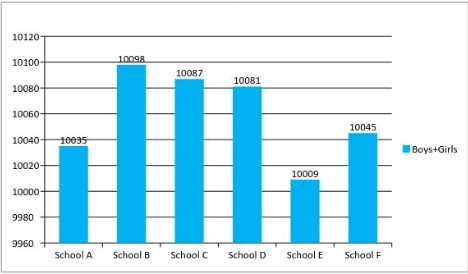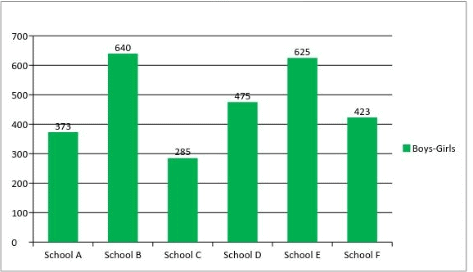What is the difference between Number of Girls in School A and Number of Girls in School B?

Detailed Solution for Test: Data Interpretation- 3 - Question 1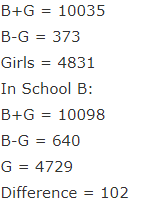Test: Data Interpretation- 3 - Question 2

### Study the following graph carefully and answer the following questions given below.Girls in School C forms approximately what percent of the total number students in that School?

Detailed Solution for Test: Data Interpretation- 3 - Question 2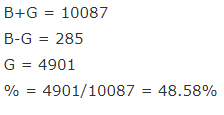Test: Data Interpretation- 3 - Question 3

### Study the following graph carefully and answer the following questions given below.What is the ratio of Sum of Boys in School D and Girls in School E together to the Sum of Girls in School D and Boys in School E together is?

Detailed Solution for Test: Data Interpretation- 3 - Question 3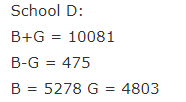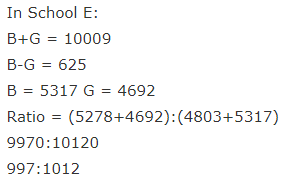Test: Data Interpretation- 3 - Question 4

Study the following graph carefully and answer the following questions given below.How many number of Boys are there in School F?

Detailed Solution for Test: Data Interpretation- 3 - Question 4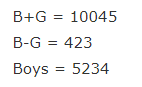Test: Data Interpretation- 3 - Question 5

Study the following graph carefully and answer the following questions given below.In which of the following School least no of Girls are present?

Detailed Solution for Test: Data Interpretation- 3 - Question 5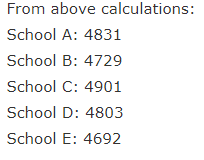Test: Data Interpretation- 3 - Question 6

Q(6-10) Study the Pie Chart and answer the following questions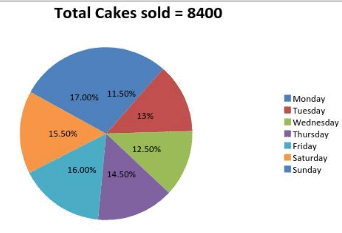The ratio of Number of Vanilla Cakes Sold to Chocolate Cakes Sold is 2:1 of the total cakes sold on Monday and the ratio of the number of Vanilla Cakes Sold to Chocolate Cakes Sold is 3:2 in the total Cakes sold on Wednesday. Then difference of Vanilla Cakes Sold on Monday and Vanilla Cakes sold on Wednesday is?

Detailed Solution for Test: Data Interpretation- 3 - Question 6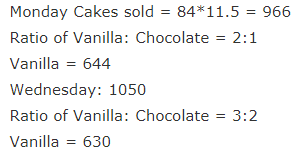Test: Data Interpretation- 3 - Question 7

Q(6-10) Study the Pie Chart and answer the following questionsIf the ratio of Vanilla Cakes Sold on Thursday to Vanilla Cakes sold on Saturday is 3:4, Number of Chocolate Cakes Sold on Thursday is equal to Number of Chocolate on Saturday then Number of Chocolate Cakes sold on Saturday is equal to total number of Cakes sold on which day?

Detailed Solution for Test: Data Interpretation- 3 - Question 7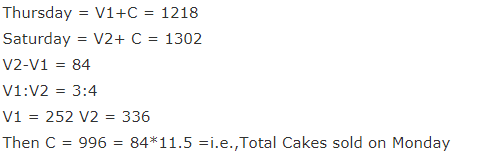Test: Data Interpretation- 3 - Question 8

Q(6-10) Study the Pie Chart and answer the following questionsIf the average number of Vanilla Cakes Sold on Friday and Sunday are 858 and Number of Chocolate Cakes Sold on Sunday are 72 more than Number of Chocolate Cakes sold on Friday then Number of Chocolate Cakes sold on Friday is?

Detailed Solution for Test: Data Interpretation- 3 - Question 8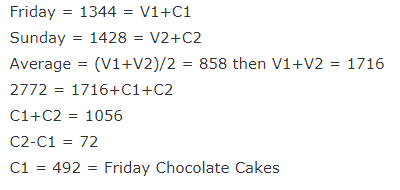Test: Data Interpretation- 3 - Question 9

Q(6-10) Study the Pie Chart and answer the following questionsRatio of Vanilla Cakes Sold to Chocolate Cakes Sold is 46:45 on Tuesday then how many number of Vanilla Cakes are Sold on that day?

Detailed Solution for Test: Data Interpretation- 3 - Question 9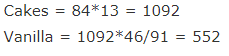Test: Data Interpretation- 3 - Question 10

Q(6-10) Study the Pie Chart and answer the following questionsIf the ratio of Vanilla Cakes sold to Chocolate Cakes sold on Monday is 2:1 and the ratio of Selling Price of Vanilla Cake to Chocolate Cake is the 1:4, total amount earned by him on Monday is Rs.9660 then what is the rate of One Vanilla Cake?

Detailed Solution for Test: Data Interpretation- 3 - Question 10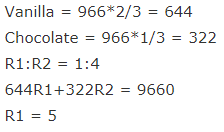Test: Data Interpretation- 3 - Question 11

Study the following graph carefully and answer the following questions given below.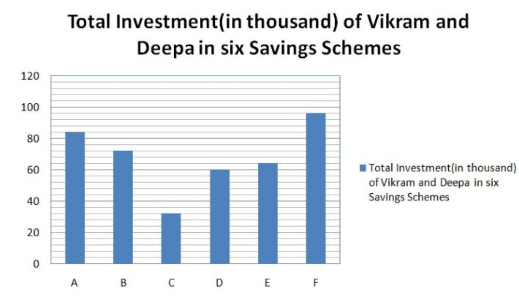The Amount invested by Vikram in savings scheme G is equal to the amount invested by him in savings scheme B. The rate of interest (p.c.p.a.) of savings scheme G and B are the same. The only difference is that savings scheme G offers compound interest (compounded annually)whereas the savings scheme B offers simple interest. If the difference between the interest earned by Vikram from both the schemes after 2 years is Rs. 349.92, what is the rate of interest?

Detailed Solution for Test: Data Interpretation- 3 - Question 11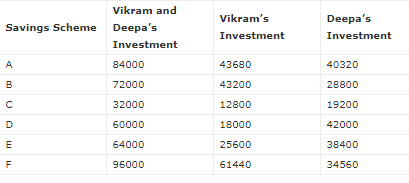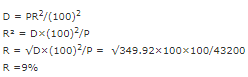Test: Data Interpretation- 3 - Question 12

Study the following graph carefully and answer the following questions given below.Deepa invested in savings scheme F for 4 years. If the savings scheme F offers simple interest at the rate of 7 p.c.p.a. for the first two years and then compound interest at the rate of 10 p.c.p.a. (compounded annually) for the third and fourth year, what will be the interest earned by Deepa after 4 years?
A. 12,364

Detailed Solution for Test: Data Interpretation- 3 - Question 12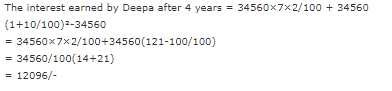Test: Data Interpretation- 3 - Question 13

Study the following graph carefully and answer the following questions given below.What is the respective ratio between the total amount invested by Vikram in scheme C and E together and the total amount invested by Deepa in the same savings scheme together?

Detailed Solution for Test: Data Interpretation- 3 - Question 13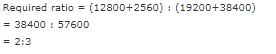Test: Data Interpretation- 3 - Question 14

Study the following graph carefully and answer the following questions given below.Savings Scheme A offers simple interest at a certain rate of interest (p.c.p.a.). If the difference between the interests earned by Vikram and Deepa from Savings Scheme A after 4 years is Rs.4435.50, what is the rate of interest (p.c.p.a)?

Detailed Solution for Test: Data Interpretation- 3 - Question 14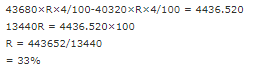Test: Data Interpretation- 3 - Question 15

Study the following graph carefully and answer the following questions given below.What is the average amount invested by Vikram in savings schemes A,B,C,D and E together?

Detailed Solution for Test: Data Interpretation- 3 - Question 15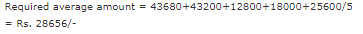Test: Data Interpretation- 3 - Question 16

Study the following graph carefully and answer the following questions given below.If Scheme C offers compound interest (compounded annually) at the rate of 12 p.c.p.a., what is the difference between interests earned by Vikram and Deepa from scheme C after 2 years?

Detailed Solution for Test: Data Interpretation- 3 - Question 16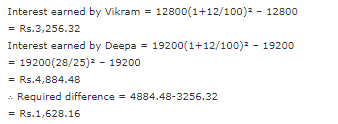Test: Data Interpretation- 3 - Question 17

Study the following graph carefully and answer the following questions given below.If the savings scheme D offers compound interest (compounded half yearly) at the rate of 16 p.c.p.a, what would be sum of interests earned by Vikram and Deepa from savings scheme D after one year?

Detailed Solution for Test: Data Interpretation- 3 - Question 17

Sum of interests earned by Vikram and Deepa = 60000(1+8/100)²-60000
= 60000(27/25)²-60000
= 60000(729-625)/625
= 60000×104/625 = Rs. 9,984/-

Test: Data Interpretation- 3 - Question 18

Study the following graph carefully and answer the following questions given below.Total investment in Savings Schemes A and F by Vikram is what percent of the total investment in the same Savings Schemes by Deepa?

Detailed Solution for Test: Data Interpretation- 3 - Question 18

Total investment in Savings Schemes A and F by Vikram = 105120
Total investment in Savings Schemes A and F by Deepa = 74880 ; 105120/74880 * 100 = 140%

Test: Data Interpretation- 3 - Question 19

Study the following graph carefully and answer the following questions given below.What is the sum of the average amount invested in Savings schemes A, D and E by Vikram and the average amount invested in Savings schemes B, C and F by Deepa?

Detailed Solution for Test: Data Interpretation- 3 - Question 19

Average amount invested in schemes A, D and E by Vikram = (43680 + 18000 + 25600)/3 = 87280/3 = 29093
Average amount invested in schemes B, C and F by Deepa = (28800 + 19200 + 34560)/3 = 82560/3 = 27520
Sum = 29093 + 27520 = 56613

Test: Data Interpretation- 3 - Question 20

Study the following graph carefully and answer the following questions given below.What is the difference between the average amount invested in Savings schemes A, B, D and E by Vikram and the average amount invested in Savings schemes B, C, E and F by Deepa?

Detailed Solution for Test: Data Interpretation- 3 - Question 20

Average amount invested in schemes A, B, D and E by Vikram = (43680 + 43200 + 18000 + 25600)/4 = 90240/4 = 32620
Average amount invested in schemes B, C, E and F by Deepa = (28800 + 19200 + 38400 + 34560)/4 = 119680/4 = 30240
Difference = 32620 – 30240 = 2380

## Quantitative Techniques for CLAT

46 videos|69 docs|95 tests
Information about Test: Data Interpretation- 3 Page
In this test you can find the Exam questions for Test: Data Interpretation- 3 solved & explained in the simplest way possible. Besides giving Questions and answers for Test: Data Interpretation- 3, EduRev gives you an ample number of Online tests for practice

## Quantitative Techniques for CLAT

46 videos|69 docs|95 tests